Documentation

# dsp.LowpassFilter

FIR or IIR lowpass filter

## Description

The `dsp.LowpassFilter` object independently filters each channel of the input over time using the given design specifications. You can set the `FilterType` property of `dsp.LowpassFilter` to `'FIR'` or `'IIR'` to implement the object as a FIR or IIR lowpass filter.

To filter each channel of your input:

1. Create the `dsp.LowpassFilter` object and set its properties.

2. Call the object with arguments, as if it were a function.

## Creation

### Syntax

``LPF = dsp.LowpassFilter``
``LPF = dsp.LowpassFilter(Name,Value)``

### Description

````LPF = dsp.LowpassFilter` returns a minimum order FIR lowpass filter, `LPF`, with the default filter settings. Calling the object with the default property settings filters the input data with a passband frequency of `8` kHz, a stopband frequency of `12` kHz, a passband ripple of `0.1` dB, and a stopband attenuation of `80` dB.```

example

````LPF = dsp.LowpassFilter(Name,Value)` returns a lowpass filter, with additional properties specified by one, or more `Name,Value` pair arguments. `Name` is the property name and `Value` is the corresponding value. `Name` must appear inside single quotes (' '). You can specify several name-value pair arguments in any order as `Name1,Value1,...,NameN,ValueN`.```

## Properties

expand all

Unless otherwise indicated, properties are nontunable, which means you cannot change their values after calling the object. Objects lock when you call them, and the `release` function unlocks them.

If a property is tunable, you can change its value at any time.

Input sample rate in Hz, specified as the comma-separated pair consisting of `'SampleRate'` and a real positive scalar.

Data Types: `single` | `double`

Filter type, specified as one of the following options:

• `'FIR'` — The object designs an FIR lowpass filter.

• `'IIR'` — The object designs an IIR lowpass (biquad) filter.

Minimum order filter design, specified as the comma-separated pair consisting of `'DesignForMinimumOrder'` and a logical value. If this property is `true`, then `dsp.LowpassFilter` designs filters with the minimum order that meets the passband frequency, stopband frequency, passband ripple, and stopband attenuation specifications. Set these specifications using the corresponding properties. If this property is `false`, then the object designs filters with the order that you specify in the `FilterOrder` property. This filter design meets the passband frequency, passband ripple, and stopband attenuation specifications that you set using the respective properties.

Order of the FIR or IIR filter, specified as the comma-separated pair consisting of `'FilterOrder'` and a positive integer scalar.

#### Dependencies

Specifying a filter order is only valid when the value of `'DesignForMinimumOrder'` is `false`.

Data Types: `single` | `double` | `int8` | `int16` | `int32` | `int64` | `uint8` | `uint16` | `uint32` | `uint64`

Filter passband edge frequency in Hz, specified as the comma-separated pair of `'PassbandFrequency'` and a real positive scalar. The value of the passband edge frequency in Hz must be less than half the `SampleRate`.

Data Types: `single` | `double` | `int8` | `int16` | `int32` | `int64` | `uint8` | `uint16` | `uint32` | `uint64`

Filter stopband edge frequency in Hz, specified as the comma-separated pair consisting of `'StopbandFrequency'` and a real positive scalar. The value of the stopband edge frequency in Hz must be less than half the `SampleRate`.

#### Dependencies

You can specify the stopband edge frequency only when `'DesignForMinimumOrder'` is `true`.

Data Types: `single` | `double` | `int8` | `int16` | `int32` | `int64` | `uint8` | `uint16` | `uint32` | `uint64`

Maximum ripple of filter response in the passband, in dB, specified as the comma-separated pair consisting of `'PassbandRipple'` and a real positive scalar. Maximum ripple of filter response defaults to `0.1` dB.

Data Types: `single` | `double` | `int8` | `int16` | `int32` | `int64` | `uint8` | `uint16` | `uint32` | `uint64`

Minimum attenuation in the stopband in dB, specified as the comma-separated pair consisting of `'StopbandAttenuation'` and a real positive scalar. Minimum attenuation in the stopband defaults to `80` dB.

Data Types: `single` | `double` | `int8` | `int16` | `int32` | `int64` | `uint8` | `uint16` | `uint32` | `uint64`

#### Fixed-Point Properties

Rounding method for output fixed-point operations, specified as a character vector. For more information on the rounding modes, see Precision and Range.

Word and fraction lengths of coefficients, specified as a `numerictype` object. The default, `numerictype(1,16)` corresponds to a signed numeric type object with 16-bit coefficients and a fraction length determined based on the coefficient values, to give the best possible precision.

This property is not tunable.

Word length of the output is same as the word length of the input. Fraction length of the output is computed such that the entire dynamic range of the output can be represented without overflow. For details on how the fraction length of the output is computed, see Fixed-Point Precision Rules for Avoiding Overflow in FIR Filters.

## Usage

### Syntax

``y = LPF(x)``

### Description

example

````y = LPF(x)` lowpass filters the input signal, `x`. `y` is a lowpass-filtered version of `x`.```

### Input Arguments

expand all

Noisy data input, specified as a vector or a matrix. If the input signal is a matrix, each column of the matrix is treated as an independent channel. The number of rows in the input signal denote the channel length. This object accepts variable-size inputs. After the object is locked, you can change the size of each input channel, but you cannot change the number of channels.

Data Types: `single` | `double` | `int8` | `int16` | `int32` | `int64` | `uint8` | `uint16` | `uint32` | `uint64` | `fi`
Complex Number Support: Yes

### Output Arguments

expand all

Filtered output, returned as a vector or a matrix. The output has the same size, data type, and complexity characteristics as the input.

Data Types: `single` | `double` | `int8` | `int16` | `int32` | `int64` | `uint8` | `uint16` | `uint32` | `uint64` | `fi`
Complex Number Support: Yes

## Object Functions

To use an object function, specify the System object™ as the first input argument. For example, to release system resources of a System object named `obj`, use this syntax:

`release(obj)`

expand all

 `freqz` Frequency response of filter `fvtool` Visualize frequency response of DSP filters `impz` Impulse response of discrete-time filter System object `info` Information about filter System object `coeffs` Filter coefficients `cost` Estimate cost for implementing filter System objects `grpdelay` Group delay response of discrete-time filter System object `generatehdl` Generate HDL code for quantized DSP filter (requires Filter Design HDL Coder) `measure` Measure frequency response characteristics of filter System object
 `step` Run System object algorithm `release` Release resources and allow changes to System object property values and input characteristics `reset` Reset internal states of System object

## Examples

expand all

Create a minimum-order FIR lowpass filter for data sampled at 44.1 kHz. Specify a passband frequency of 8 kHz, a stopband frequency of 12 kHz, a passband ripple of 0.1 dB, and a stopband attenuation of 80 dB.

```Fs = 44.1e3; filtertype = 'FIR'; Fpass = 8e3; Fstop = 12e3; Rp = 0.1; Astop = 80; FIRLPF = dsp.LowpassFilter('SampleRate',Fs, ... 'FilterType',filtertype, ... 'PassbandFrequency',Fpass, ... 'StopbandFrequency',Fstop, ... 'PassbandRipple',Rp, ... 'StopbandAttenuation',Astop);```

Design a minimum-order IIR lowpass filter with the same properties as the FIR lowpass filter. Change the `FilterType` property of the cloned filter to `IIR`.

```IIRLPF = clone(FIRLPF); IIRLPF.FilterType = 'IIR';```

Plot the impulse response of the FIR lowpass filter. The zeroth-order coefficient is delayed by 19 samples, which is equal to the group delay of the filter. The FIR lowpass filter is a causal FIR filter.

`fvtool(FIRLPF,'Analysis','impulse')`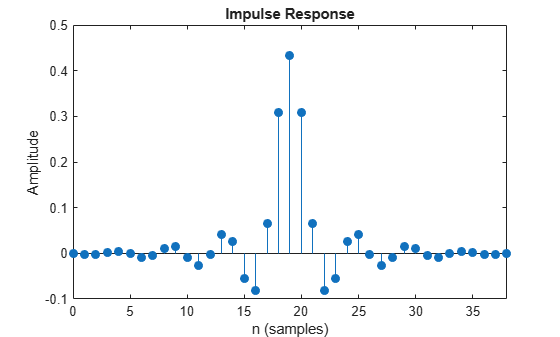Plot the impulse response of the IIR lowpass filter.

`fvtool(IIRLPF,'Analysis','impulse')`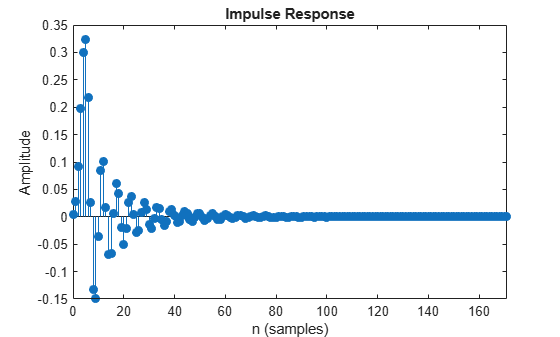Plot the magnitude and phase response of the FIR lowpass filter.

`fvtool(FIRLPF,'Analysis','freq')`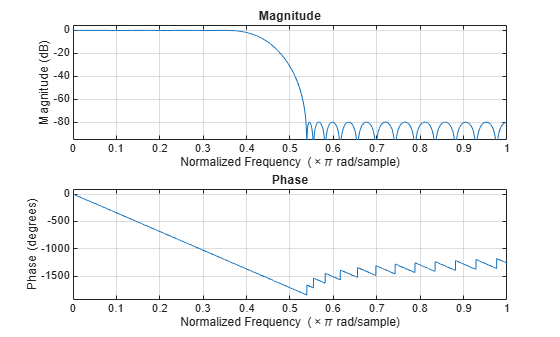Plot the magnitude and phase response of the IIR lowpass filter.

`fvtool(IIRLPF,'Analysis','freq')`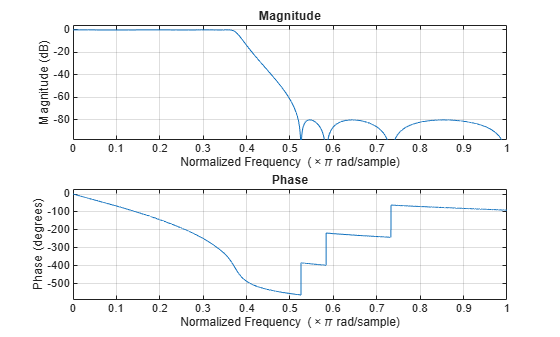Calculate the cost of implementing the FIR lowpass filter.

`cost(FIRLPF)`
```ans = struct with fields: NumCoefficients: 39 NumStates: 38 MultiplicationsPerInputSample: 39 AdditionsPerInputSample: 38 ```

Calculate the cost of implementing the IIR lowpass filter. The IIR filter is more efficient to implement than the FIR filter.

`cost(IIRLPF)`
```ans = struct with fields: NumCoefficients: 18 NumStates: 14 MultiplicationsPerInputSample: 18 AdditionsPerInputSample: 14 ```

Calculate the group delay of the FIR lowpass filter.

`grpdelay(FIRLPF)`Calculate the group delay of the IIR lowpass filter. The FIR filter has a constant group delay (linear phase), while its IIR counterpart does not.

`grpdelay(IIRLPF)`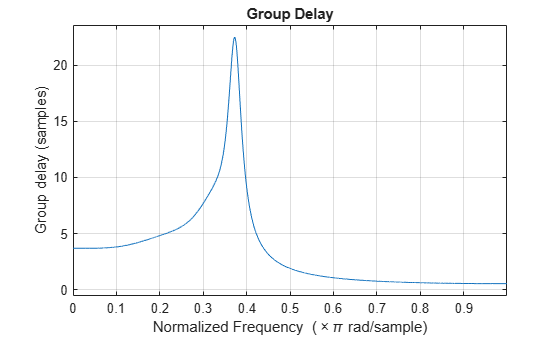Create a lowpass filter with default properties.

```LPF = dsp.LowpassFilter; ```

Create a spectrum analyzer object.

```hSA = dsp.SpectrumAnalyzer('SampleRate',44.1e3,... 'PlotAsTwoSidedSpectrum',false,'ShowLegend',true,'YLimits',... [-150 30],... 'Title',... 'Input Signal and Output Signal of Lowpass Filter'); hSA.ChannelNames = {'Input','Output'}; ```

Implement `step` on `LPF` to filter the white Gaussian noisy input signal. View the input and output signals using the spectrum analyzer.

```for k = 1:100 Input = randn(1024,1); Output = step(LPF,Input); step(hSA,[Input,Output]); end ```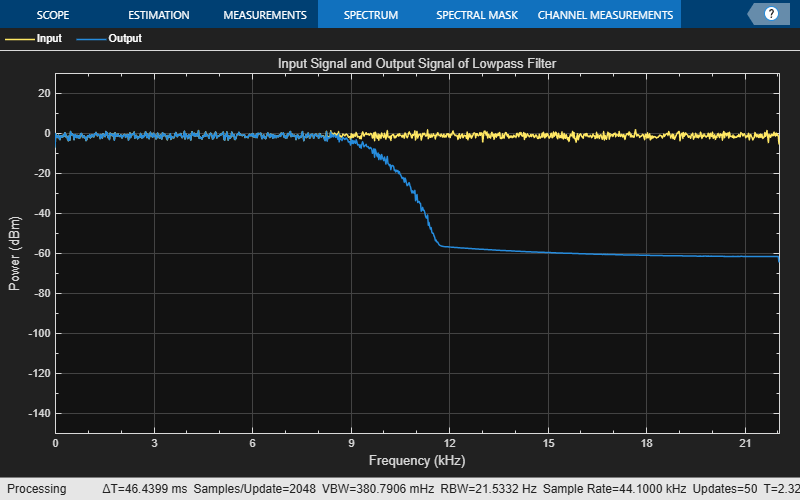Note: This example runs only in R2016b or later. If you are using an earlier release, replace each call to the function with the equivalent `step` syntax. For example, myObject(x) becomes step(myObject,x).

Set up the IIR lowpass filter. The sampling rate of the white Gaussian noise is 44,100 Hz. The passband frequency of the filter is 8 kHz, the stopband frequency is 12 kHz, the passband ripple is 0.1 dB, and the stopband attenuation is 80 dB.

```Fs = 44.1e3; filtertype = 'IIR'; Fpass = 8e3; Fstop = 12e3; Rp = 0.1; Astop = 80; LPF = dsp.LowpassFilter('SampleRate',Fs,... 'FilterType',filtertype,... 'PassbandFrequency',Fpass,... 'StopbandFrequency',Fstop,... 'PassbandRipple',Rp,... 'StopbandAttenuation',Astop); ```

View the magnitude response of the lowpass filter.

```fvtool(LPF) ```Create a spectrum analyzer object.

```hSA = dsp.SpectrumAnalyzer('SampleRate',44.1e3,... 'PlotAsTwoSidedSpectrum',false,'ShowLegend',true,'YLimits',... [-150 30],... 'Title',... 'Input Signal and Output Signal of IIR Lowpass Filter'); hSA.ChannelNames = {'Input','Output'}; ```

Filter the white Gaussian noisy input signal. View the input and output signals using the spectrum analyzer.

```for k = 1:100 Input = randn(1024,1); Output = LPF(Input); hSA([Input,Output]); end ```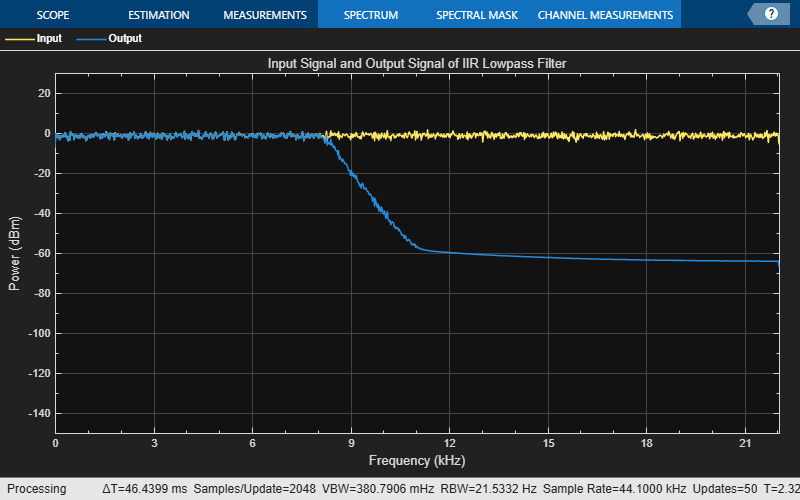Measure the frequency response characteristics of a lowpass filter. Create a `dsp.LowpassFilter` System object with default properties. Measure the frequency response characteristics of the filter.

`LPF = dsp.LowpassFilter`
```LPF = dsp.LowpassFilter with properties: FilterType: 'FIR' DesignForMinimumOrder: true PassbandFrequency: 8000 StopbandFrequency: 12000 PassbandRipple: 0.1000 StopbandAttenuation: 80 SampleRate: 44100 Show all properties ```
`LPFMeas = measure(LPF)`
```LPFMeas = Sample Rate : 44.1 kHz Passband Edge : 8 kHz 3-dB Point : 9.1311 kHz 6-dB Point : 9.5723 kHz Stopband Edge : 12 kHz Passband Ripple : 0.08289 dB Stopband Atten. : 81.6141 dB Transition Width : 4 kHz ```

## Algorithms

expand all

 Shpak, D.J., and A. Antoniou. "A generalized Remez method for the design of FIR digital filters." IEEE® Transactions on Circuits and Systems. Vol. 37, Issue 2, Feb. 1990, pp. 161–174.

 Selesnick, I.W., and C. S. Burrus. "Exchange algorithms that complement the Parks-McClellan algorithm for linear-phase FIR filter design." IEEE Transactions on Circuits and Systems. Vol. 44, Issue 2, Feb. 1997, pp. 137–143.

Watch now